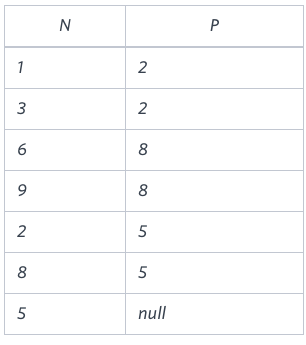# How to use NOT IN in binary tree problem using MySQL

I am trying to solve this problem,

You are given a table, BST, containing two columns: N and P, where N
represents the value of a node in Binary Tree, and P is the parent of
N.

Write a query to find the node type of Binary Tree ordered by the
value of the node. Output one of the following for each node:

```Root: If node is root node.
Leaf: If node is leaf node.
Inner: If node is neither root nor leaf node.```

Input:Desired Output:

```1 Leaf
2 Inner
3 Leaf
5 Root
6 Leaf
8 Inner
9 Leaf```

This is my query, can anyone tell me why it’s not working?

```select case
when P is NULL then CONCAT_WS(" ", N, 'Root')
when N not in (SELECT DISTINCT P FROM BST) then CONCAT_WS(" ", N, 'Leaf')
else CONCAT_WS(" ", N, 'Inner')
end
from BST ORDER BY N ASC;```
Contents

### Method 1

You have `NULL` inside the `P` column in which case an expression such as:

`1 NOT IN (NULL, 2, 8, 5)`

will return `unknown` instead of the “expected” result `true` (ref).

The solution is to make a subtle change like so:

`N NOT IN (SELECT P FROM BST WHERE P IS NOT NULL)`

Or use an `EXISTS` query:

`NOT EXISTS (SELECT * FROM BST AS bst2 WHERE bst2.P = bst.N)`

### Method 2

``````SELECT n,
ELT((1 + (2 * (t1.p IS NULL)) + (EXISTS (SELECT NULL FROM BST t2 WHERE t1.n=t2.p))), 'Leaf', 'Inner', 'Single', 'Root') type
FROM BST t1
ORDER BY n;
``````

https://dbfiddle.uk/?rdbms=mysql_8.0&fiddle=287b1de2b2bb532d73619c19bcf8a86b

I add one more option ‘Single’ – the case when the node is root and leaf at the same time (node 4 in my fiddle).